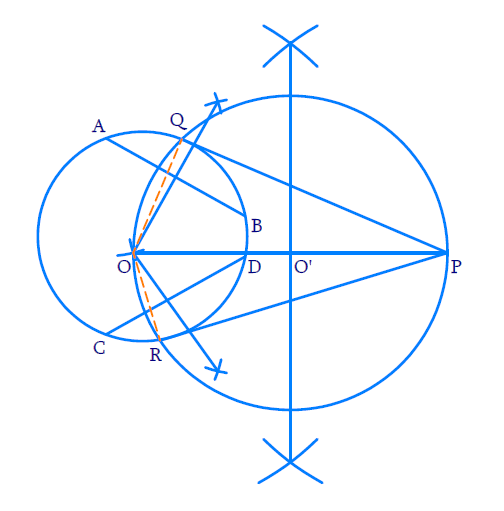# Ex.11.2 Q7 Constructions Solution - NCERT Maths Class 10

Go back to  'Ex.11.2'

## Question

Draw a circle with the help of a bangle. Take a point outside the circle. Construct the pair of tangents from this point to the circle.

Video Solution
Constructions
Ex 11.2 | Question 7

## Text Solution

Steps:Steps of construction:

(i)  Draw any circle using a bangle.

To find its centre

(a) Draw any two chords of the circle say $$AB$$ and $$CD$$.

(b) Draw the perpendicular bisectors of $$AB$$ and $$CD$$ to intersect at $$O$$.

Now, $$‘O’$$ is the centre of this circle (since the perpendiculars drawn from the centre of a circle to any chord bisect the chord and vice versa).

To draw the tangents from a point $$‘P’$$ outside the circle.

(ii) Take any point $$P$$ outside the circle and draw the perpendicular bisector of $$OP$$ which meets at $$OP$$ at $$O’$$.

(iii) With $$O’$$ as center and $$OO’$$ as radius draw a circle which cuts the given circle at $$Q$$ and $$R$$.

(iv) Join $$PQ$$ and $$PR$$.

$$PQ$$ and $$PR$$ are the required tangents.

Proof:

$$\angle {\rm{OQP}} = \angle {\rm{ORP}} = 90^\circ$$ (Angle in a semi $$-$$ circle)

$$\therefore \,{OQ} \,\bot\, {QP}$$ and $${OR}\, \bot\, {RP}$$

Hence, $$PQ$$ and $$PR$$ are the tangents to the given circle.

Learn from the best math teachers and top your exams

• Live one on one classroom and doubt clearing
• Practice worksheets in and after class for conceptual clarity
• Personalized curriculum to keep up with school